# Visual Tracking Robot

ECE 5725 Final Project (SP2022) by
Honglei Huo
Fanghan Li

## Introduction

Robotics is the future. Robot-human interaction can be applied widely.This project is a simple exploration of this interaction.
The whole project is divided into two parts: image processing and servo control. The image part is based on opencv and python; the camera can detect the concours of objects in the frame. Then the position and size of the contours will be calculated and send different commands to the GPIO pins. The control is dominated by a microcontroller. Based on the low and high levels of output, the robot can take actions accordingly.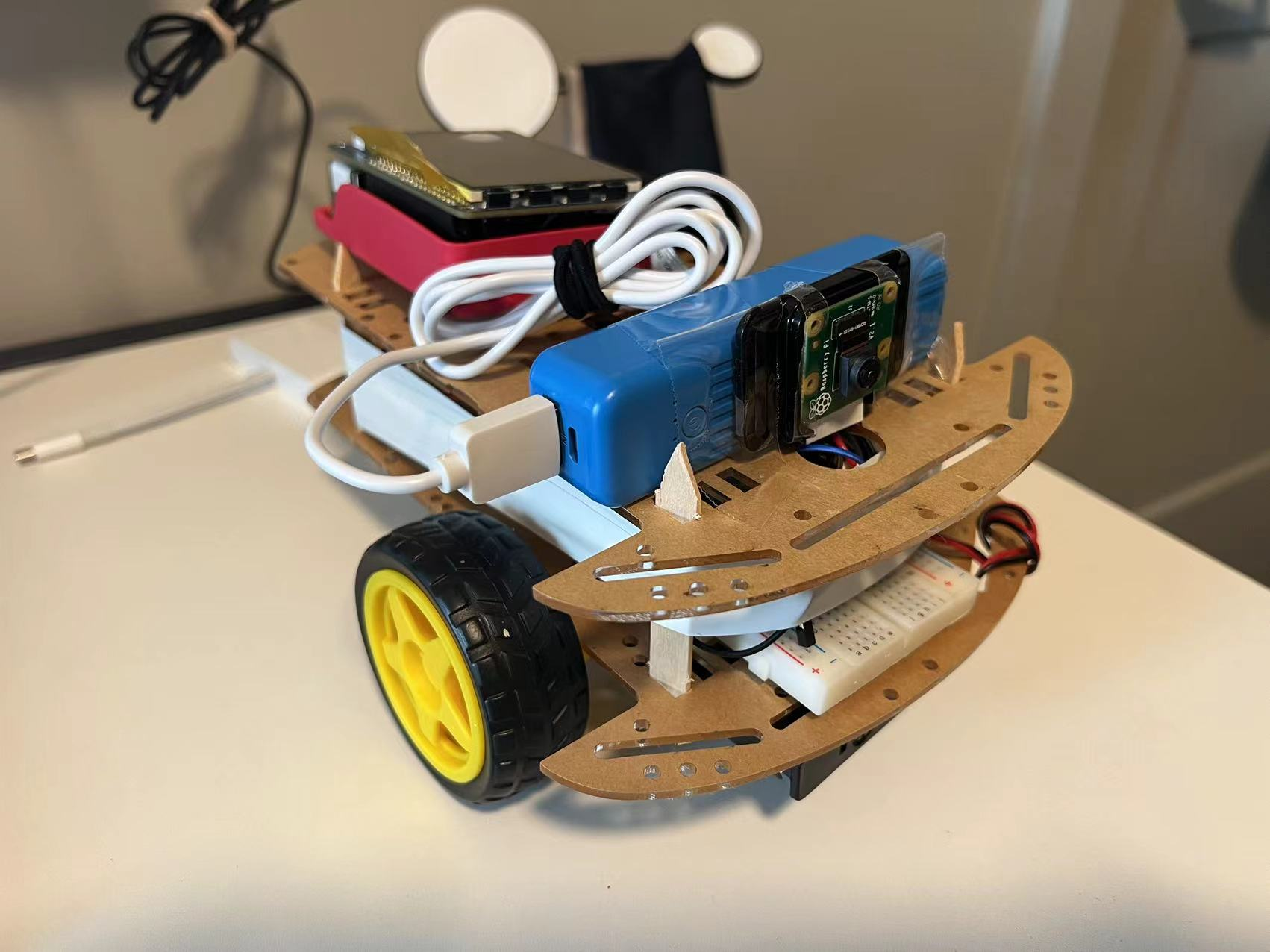## Design & Testing

Software:

The first step is to turn on the Pi camera to capture the frames.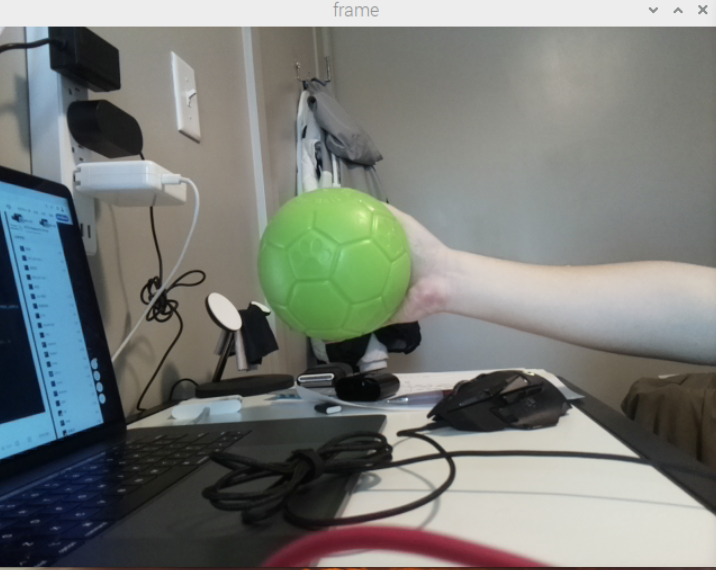###### Frame captured by Pi camera

Then I used the Gaussian filter to remove noise; make colors on the frame more highlighted and easier to be detected.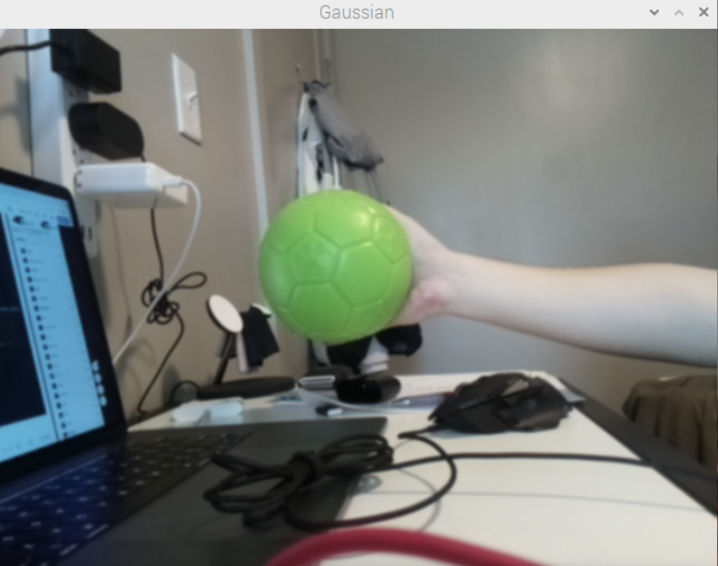###### Image processed by Gaussian filter

Then transfer the colors from BGR (Blue, Green, Red) color space to HSV (Hue, Saturation, Value). Since the colors in HSV are easier to be figured out by the computer. The next step is to set a threshold value to remove all other colors, which are on the outside of the threshold, only the green part will remain. At this moment, the content of the frame is a black background, a large white area and some white points of noise. The shape of the white area is the shape of the green object being detected.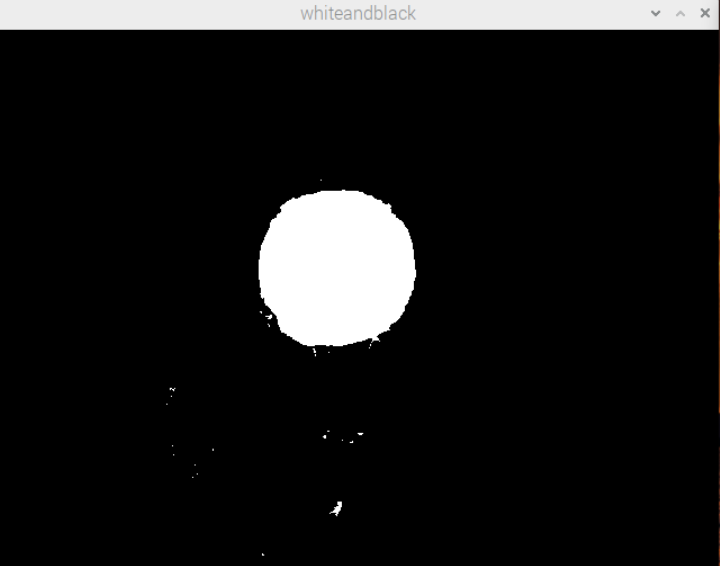###### Image only remain the green object

However, for now, the boundaries are not clear, with some tiny burrs on the boundary. There are also some noise points on the frame. So I used 3x3 kernels to erode the frame for 2 iterations before detecting the contours.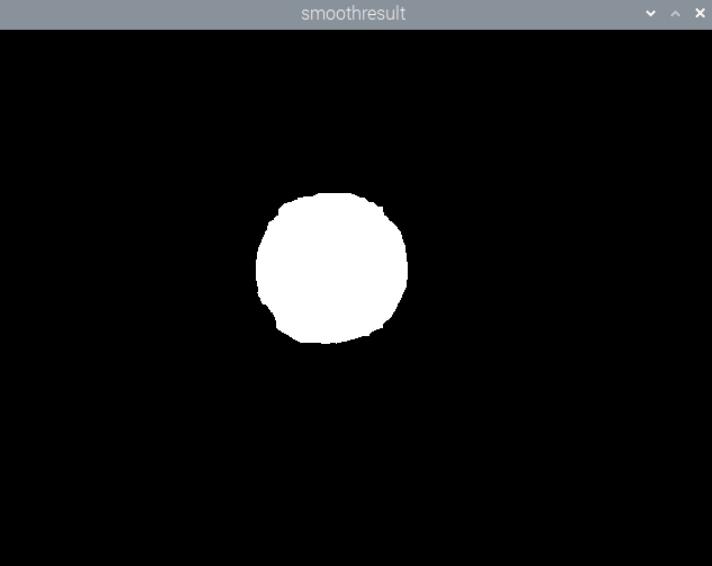###### Image after erode

The reason I chose 3x3 kernels and 2 iterations is I want to make the detection more accurate and remove noise, but I do not want to make the white area too small.

Now, a circumcircle can be drawn based on the contour. For the contour, I chose RETR_EXTERNAL mode and CHAIN_APPROX_SIMPLE method to reduce the computing cost. The information of the circle (coordination of the center of the circle and radius) will be sent to the control part.

At the beginning of the program, I set some constants as x_mid = 320 ( since the size of the frame is 640x480), x_bias = 130. So the frame can be divided into 3 parts shown in the following figure.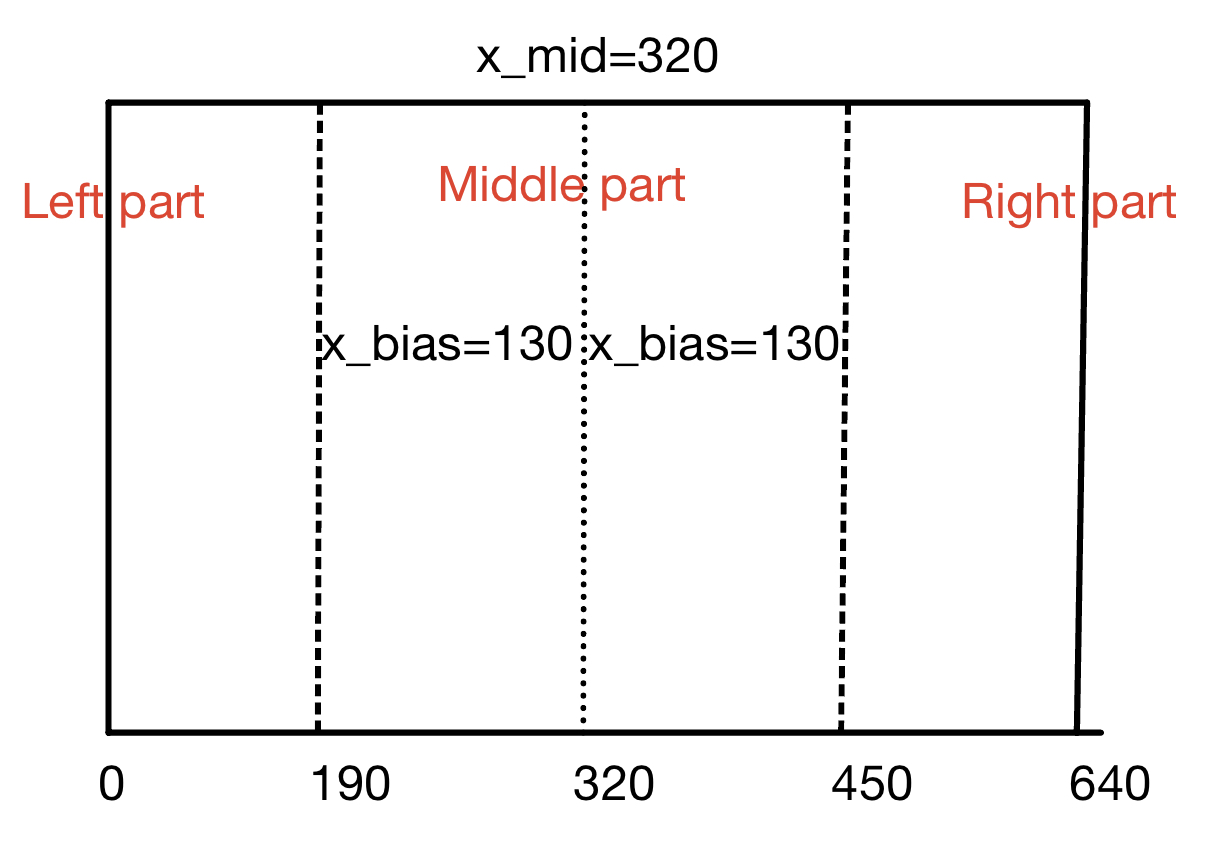###### Deviding the frame in to 3 parts

Once the green ball appears in the right part, the robot will turn to the right to make the ball get into the middle part. Since the operating system is not real-time. There is always some legacy between data collection, computation and action. So I set a sleep time as 0.05 second for the turning to avoid rotating too much and losing the target. I also set 2 constants for radius, which are rad_mid =70 and rad_bias = 30. The radius of the circumcircle changes with the distance between the robot and the green ball. If radius - rad_mid > rad_bias, the robot will go forward, if rad_mid - radius > rad_bias, robot will move backward. The information of the circumcircle and motion will be printed out on the piTFT.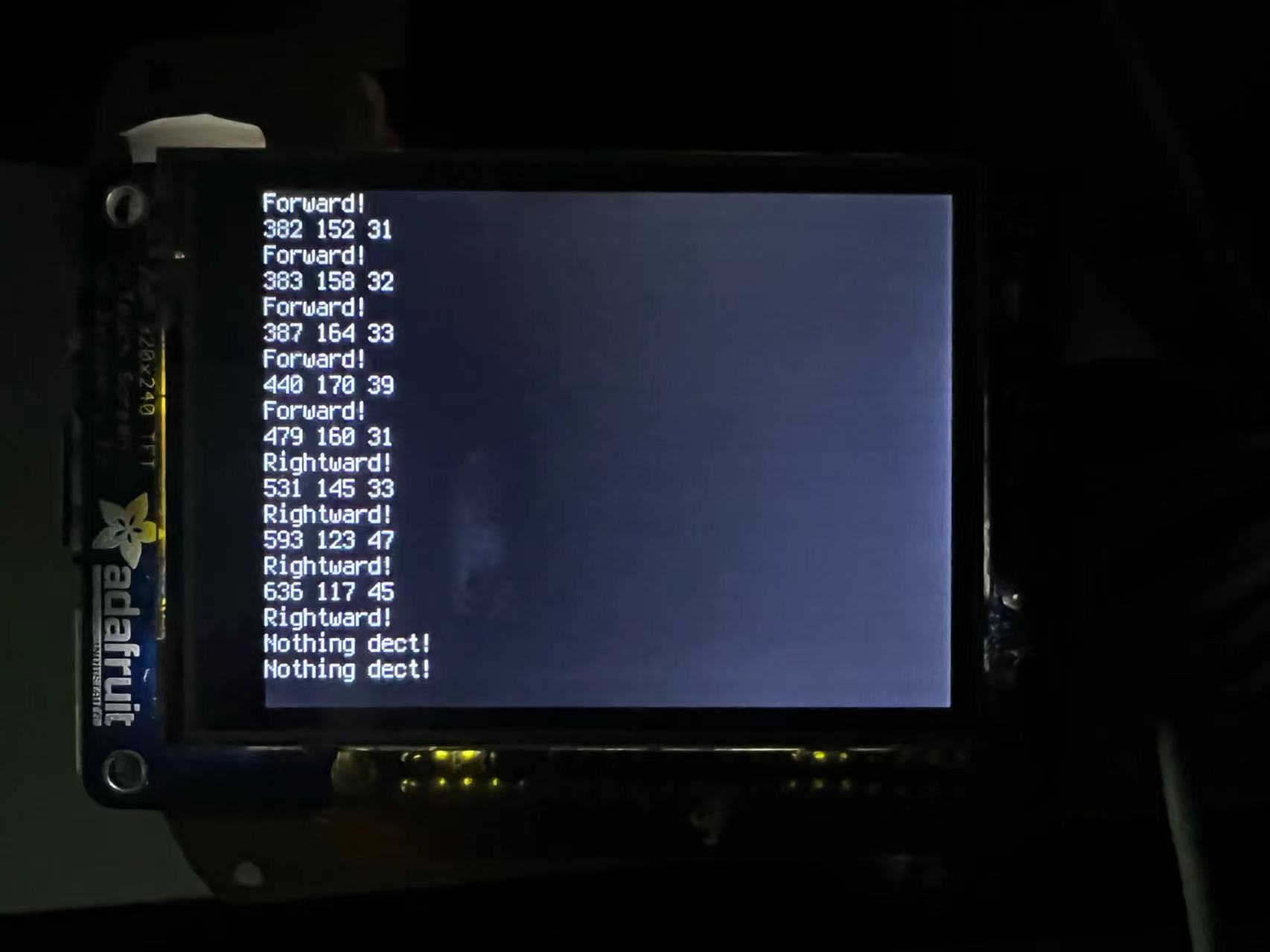###### piTFT display

There is also a flag value in the control. If there is nothing detected, or the radius is less than 20. The flag is 0, the robot will stop.

Hardware:

I mainly used a Raspberry Pi, TB6612FNG microcontroller, 2 servos, resisters, diodes and breadboard to build the dynamics system.

The schematics are shown in the following picture.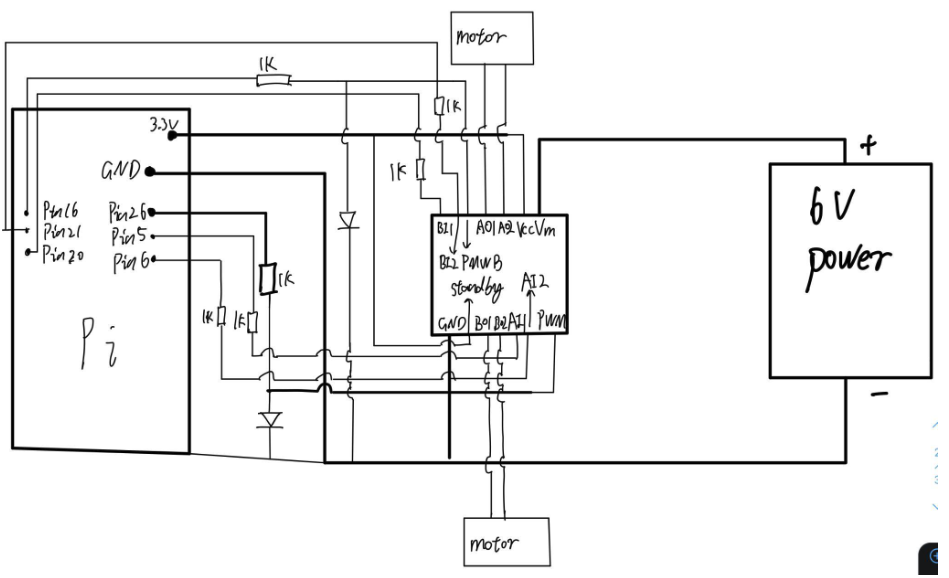###### schematics

The points should be emphasized:

1. To protect the GPIO pins, a 1k resistor should be placed between the GPIO pins and inputs of the microcontroller.
2. I used the diodes to check the working status of PWM at any time.
3. Make sure the relation between rotating direction and High/Low inputs based on the table below.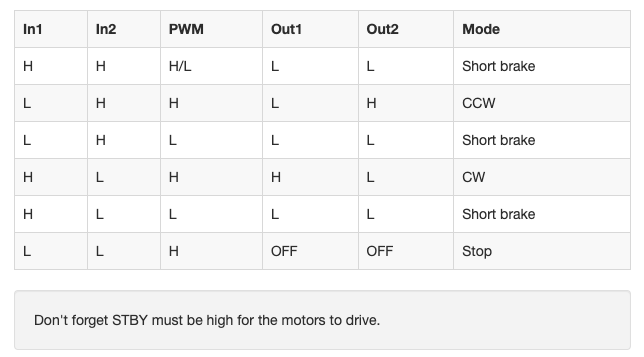## Result & Conclusion

The project was completed successfully as the proposal expected. However, it’s not as perfect as I expected. As I mentioned before, the program is running on a non-real-time operating system. So the speed of rotation is limited, if the ball runs too fast, the robot would lose the target easily.
Secondly, the robot will stay away from the ball for a certain distance, but I’m only using Opencv to do the measurement. So the distance is not an exact constant, the elements which can affect the image processing result, such as light, can also affect the distance.
During the project, I encountered many challenges, but after researching and self-learning, I’m really happy I can solve the problems.

## Future Work

This project is an attempt to explore the human-robot interaction. I believe that it could be developed into a more useful and helpful robot. For example, machine learning could be used to recognize the user, and the servos could be replaced as more powerful ones, so it could help people to carry bags or something else.

## Individual Contribution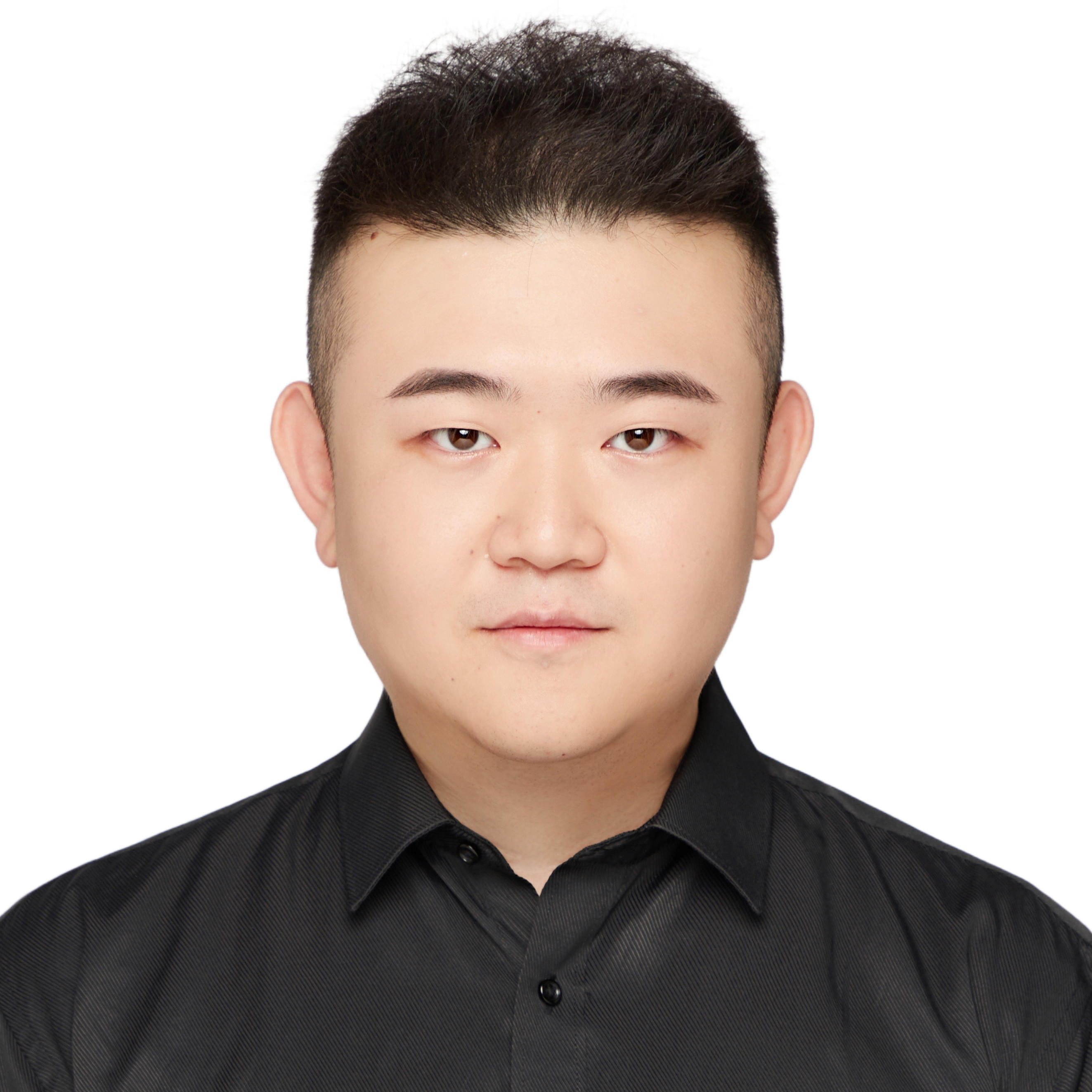### Honglei Huo

hh547@cornell.edu

Project idea generation.

Designed the overall program, algorithms and control logic.

Designed and built the circuit and robot structure.

Overall testing and debugging.

Report writing and website set up.### Fanghan Li

fl366@cornell.edu

Installed servos and wheels on the robot.

## Parts List

• Raspberry Pi \$35.00
• Raspberry Pi Camera V2 \$25.00
• TB6612FNG microcontroller - \$5.50
• Robot Kit - Provided in lab
• LEDs,Batteries, Breadboard, Resistors and Wires - Provided in lab

## References

PiCamera Document
OpenCV
TB6612FNG Hookup Guide

900-00008-Continuous-Rotation-Servo-Documentation

R-Pi GPIO Document

## Code Appendix

``````
import RPi.GPIO as GPIO
import time
import cv2
import numpy as np

cap = cv2.VideoCapture(0)

GPIO.setmode(GPIO.BCM)
GPIO.setup(21,GPIO.OUT)
GPIO.setup(26,GPIO.OUT)
GPIO.setup(5,GPIO.OUT)
GPIO.setup(6,GPIO.OUT)
GPIO.setup(16,GPIO.OUT)
GPIO.setup(12,GPIO.OUT)

x_bias = 130
x_now = 0
x_mid= 320
control_flag = 0

p1 = GPIO.PWM(26, 50)
p2 = GPIO.PWM(21, 50)
p1.start(0)
p2.start(0)

def Car_Forward():
p1.start(75)
p2.start(75)
GPIO.output(16,GPIO.HIGH)
GPIO.output(12,GPIO.LOW)
GPIO.output(5, GPIO.HIGH)
GPIO.output(6, GPIO.LOW)

def Car_Backward():
p1.start(75)
p2.start(75)
GPIO.output(16,GPIO.LOW)
GPIO.output(12,GPIO.HIGH)
GPIO.output(5, GPIO.LOW)
GPIO.output(6, GPIO.HIGH)

def Car_Leftturn():
p1.start(65)
p2.start(65)
GPIO.output(16,GPIO.HIGH)
GPIO.output(12,GPIO.LOW)
GPIO.output(5, GPIO.LOW)
GPIO.output(6, GPIO.HIGH)

def Car_Rightturn():
p1.start(65)
p2.start(65)
GPIO.output(16,GPIO.LOW)
GPIO.output(12,GPIO.HIGH)
GPIO.output(5, GPIO.HIGH)
GPIO.output(6, GPIO.LOW)

def Car_Stop():
p1.start(0)
p2.start(0)
GPIO.output(16,GPIO.LOW)
GPIO.output(12,GPIO.LOW)
GPIO.output(5, GPIO.LOW)
GPIO.output(6, GPIO.LOW)

def setup():
GPIO.setup(16, GPIO.OUT)
GPIO.setup(12, GPIO.OUT)
GPIO.setup(5, GPIO.OUT)
GPIO.setup(6, GPIO.OUT)
Car_Stop()

def run():
global x_bias
global x_now
global y_now
global control_flag

while True:
cv2.resizeWindow("frame",640,480)
########################image processing###########################
Gaussian = cv2.GaussianBlur(frame, (3, 3), 0)
hsvspace = cv2.cvtColor(Gaussian, cv2.COLOR_BGR2HSV)
green_lower = np.array([35, 43, 35])
green_upper = np.array([90, 255, 255])
whiteandblack = cv2.inRange(hsvspace, green_lower, green_upper)
kernal = np.ones((3,3))
smoothresult = cv2.erode(whiteandblack, kernal, iterations=2)
contour = cv2.findContours(smoothresult.copy(), cv2.RETR_EXTERNAL, cv2.CHAIN_APPROX_SIMPLE)[-2]

if len(contour) > 0:
area = max(contour, key=cv2.contourArea)
control_flag = 1
x_now = x
############################control##################################
if control_flag == 1:
if x_now - x_mid > x_bias:
print('Rightward!')
Car_Rightturn()
time.sleep(0.05)
Car_Stop()
elif x_mid - x_now > x_bias:
print('Leftward!')
Car_Leftturn()
time.sleep(0.05)
Car_Stop()
print('Backward!')
Car_Backward()
print('Forward!')
Car_Forward()
else:
print('Stop!')
Car_Stop()

else:
control_flag = 0
print('Nothing detected!')
Car_Stop()
else:
pass
####################For Testing########################################
#cv2.imshow('frame', frame)
#cv2.imshow('Gaussian',Gaussian)
#cv2.imshow('whiteandblack',whiteandblack)
#cv2.imshow('smoothresult',smoothresult)
####################Quit#######################################
if cv2.waitKey(5) & 0xFF == 27: #ESC
break

######################shut down everything, close windows#########################
def destroy():
Car_Stop()
p1.stop()
p2.stop()
GPIO.cleanup()
cap.release()
cv2.destroyAllWindows()

if __name__ == '__main__':
setup()
print('System Start!')
try:
run()
except KeyboardInterrupt:
destroy()
p1.stop()
p2.stop()
GPIO.cleanup()

``````